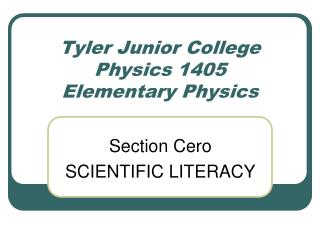# Tyler Junior College Physics 1405 Elementary Physics - PowerPoint PPT PresentationDownload PresentationTyler Junior College Physics 1405 Elementary Physics

Tyler Junior College Physics 1405 Elementary Physics
Download Presentation## Tyler Junior College Physics 1405 Elementary Physics

- - - - - - - - - - - - - - - - - - - - - - - - - - - E N D - - - - - - - - - - - - - - - - - - - - - - - - - - -
##### Presentation Transcript

1. Tyler Junior CollegePhysics 1405Elementary Physics Section Cero SCIENTIFIC LITERACY

2. Important Concepts • Measurements & Units • Your Need for Scientific Literacy • Science & Pseudoscience • Scientific Reasoning • A Brief History of Western Science

3. PHYSICS

4. Physics . . . • is the science of nature in the broadest sense. • is the science of simple things. • studies matter, energy, & the fundamental forces of nature. • deals with descriptions of the physical universe • can be divided into many fields: mechanics, electromagnetics, atomic, nuclear, astrophysics, quantum, particle, geo, etc.

5. MEASUREMENTS

6. What You Need To Bring • Pencils • Three 882-E Scantrons • 1 pkg 815-E Scantrons • Scientific Calculator • Centimeter ruler • Protractor • Pickup your clicker & return at end of class

7. Measuring Stuff • Measurements are a hallmark of good science. • How much you know about something is often related to how well you can measure it. • If we can’t quantify it, categorize, or in some way classify it, then a value cannot be placed on it.

8. Measurements • Measurements include a number and a unit. Ex: 2 cm, 14 weeks, 10 gallons. • Measurements require an agreed upon standard to be defined. Ex: meter, yard, day, gallon, kilogram, degree

9. Unit Conversion Conversion of units is an important part of working with physical quantities. Convert 27 inches to meters Convert 70 mi/hr to ft/s Convert 1 yd3 to m3

10. Fundamental Quantities • Fundamental quantities: Ex: mass, length, time, charge, luminosity • Non-fundamental quantities: Ex: area, volume, force, density, acceleration • How to tell the difference:the units of non-fundamental quantities are composed of units of fundamental quantities

11. Units Are Often Mixed • Density = mass / volume • Units of density in mks: kg/m3 • Velocity = distance / time • Units of velocity in cgs: cm/s

12. International System of Units (SI) • mks – meter, kilogram, second • cgs – centimeter, gram, second • Prefixes: • deci = 1/10 = 10-1 • centi = 1/100 = 10-2 (cm) • milli = 1/1000 = 10-3 (mm) • micro = 1/1000000 = 10-6 (m) • Many, many others in text (Know These)

13. Reading Your Text: Measuring Stuff • Notice the progression of thought • Eratosthenes measures Earth’s diameter • Aristarchus measures Moon’s diameter • Measurement of Earth-Moon distance • Measurement of Earth-Sun distance • Measurement of Sun’s diameter • Also notice no special tools required • You could have done this!

14. YOUR NEED FOR SCIENTIFIC LITERACY

15. Scientific literacy… …is knowledge and understanding of the scientific concepts and processes required for personal decision making, participation in civic and cultural affairs, and economic productivity (National Academy of Sciences, 1995).

16. Closing the Gaps • The reasoning behind CTG…. • High tech jobs have high pay. • High tech jobs require strong understanding of scientific principles. • Therefore, if you want high pay, you need to possess scientific understanding

17. Education equals More \$\$\$

18. Test Your Scientific Literacy Now let’s take a scientific literacy test. Keep your score.

19. Answer each question with 'true' if what the sentence most normally means is typically true and 'false' if it is typically false. 1. Scientists usually expect an experiment to turn out a certain way. 2. Science only produces tentative conclusions that can change. 3. Science has one uniform way of conducting research called “the scientific method.” 4. Scientific theories are explanations and not facts. 5. When being scientific one must have faith only in what is justified by empirical evidence. 6. Science is just about the facts, not human interpretations of them. 7. To be scientific one must conduct experiments. 8. Scientific theories only change when new information becomes available. 9. Scientists manipulate their experiments to produce particular results. 10. Science proves facts true in a way that is definitive and final. 11. An experiment can prove a theory true. 12. Science is partly based on beliefs, assumptions, and the nonobservable. 13. Imagination and creativity are used in all stages of scientific investigations.

20. 14. Scientific theories are just ideas about how something works. 15. A scientific law is a theory that has been extensively and thoroughly confirmed. 16. Scientists’ education, background, opinions, disciplinary focus, and basic guiding assumptions and philosophies influence their perception and interpretation of the available data. 17. A scientific law will not change because it has been proven true. 18. An accepted scientific theory is a hypothesis that has been confirmed by considerable evidence and has endured all attempts to disprove it. 19. A scientific law describes relationships among observable phenomena but does not explain them. 20. Science relies on deduction (x entails y) more than induction (x implies y). 21. Scientists invent explanations, models or theoretical entities. 22. Scientists construct theories to guide further research. 23. Scientists accept the existence of theoretical entities that have never been directly observed. 24. Scientific laws are absolute or certain.

21. 25. • Would you buy Penta water? • http://www.pentawater.com/index.php